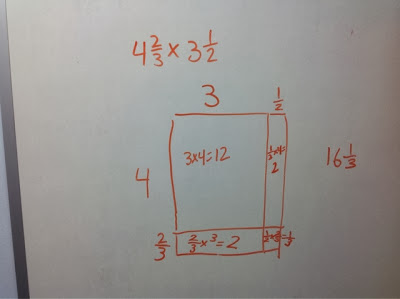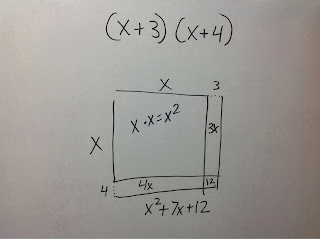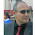## Wednesday, November 13, 2013

### Using the Area Model to Teach Mixed Number Multiplication

Have you started using the area model to teach multi-digit multiplication?  How about using it to teach fraction or mixed number multiplication?Here is an example of using the area model to teach double digit multiplication.  I do this in fourth grade with students and it really helps them develop their conceptual understanding.  It really helps students see why you can't just multiply the tens with the tens and the ones with the ones.   If students are exposed to this model in grade 4, it is a natural extension to use the area model to teach fraction multiplicationHere is the area model for mixed numbers.  Again it really helps kids see why you can't just multiply the whole numbers together and the fractions together.  It is also very efficient and involves less computation (and therefore room for error) than the traditional algorithm for fraction multiplication.  As you can see, students must be comfortable multiplying two fractions less than 1 and a fraction by a whole number.Here is the same problem done with a standard algorithm.  See how little fraction understanding goes into this way and how much computation takes place?  I find once my students know both methods, they pick and choose which to use based on efficiency which is always a great thing!If you are looking for further evidence that the area model can be a great conceptual tool for students learning multiplication, check out how well it works in an algebra classroom.  Instead of teaching kids to just FOIL, you can connect their new learning to the knowledge they already have about multiplication.
How do you use the area model when teaching multiplication?  Respond in the comments below!

1.2.3.